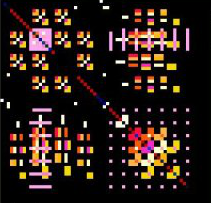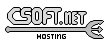<-- Back to AG_Intro.3

# SYNOPSIS

 ```#include #include #include ```

# DESCRIPTIONThe M_Vector(3) and M_Matrix interfaces implement linear algebra operations on (real or complex valued) n dimensional vectors, and m by n matrices. Optimized interfaces are provided for fixed-dimensional types (which have entries directly accessible as x, y, z and w). Arbitrary-dimensional types may or may not use fixed arrays in memory. For example, the "sparse" backend uses a sparse matrix representation, and the "db" backend stores vector entries in a database. Backends can be selected at run-time, or Agar-Math can be compiled to provide inline expansions of all operations of a specific backend. Vector extensions (such as SSE and AltiVec) are used by default, if a runtime cpuinfo check determines that they are available (the build remains compatible with non-vector platforms, at the cost of extra function calls). For best performance, Agar should be compiled with "--with-sse=inline", or "--with-altivec=inline".

# M-BY-N MATRICES

The following routines operate on dynamically-allocated m by n matrices:
 fpu Native scalar floating point methods. sparse Methods optimized for large, sparse matrices. Based on the excellent Sparse 1.4 package by Kenneth Kundert at UC Berkeley Pq Lk http://sparse.sourceforge.net/ .

# M-BY-N MATRICES: INITIALIZATION

 M_Matrix * M_New (Uint m, Uint n)void M_Free (M_Matrix *M)int M_Resize (M_Matrix *M, Uint m, Uint n)void M_SetIdentity (M_Matrix *M)void M_SetZero (M_Matrix *M)int M_Copy (M_Matrix *D, const M_Matrix *A)M_Matrix * M_Dup (const M_Matrix *M)M_Matrix * M_ReadMatrix (AG_DataSource *ds)void M_WriteMatrix (AG_DataSource *ds, const M_Matrix *A)

The M_New() function allocates a new m by n matrix. M_Free() releases all resources allocated for the specified matrix. M_Resize() resizes M to m by n. Existing entries are preserved, but new entries are left uninitialized. If insufficient memory is available, -1 is returned and an error message is set. On success, the function returns 0.

M_SetIdentity() initializes M to the identity matrix. M_SetZero() initializes M to all zeros.

M_Copy() copies the contents of matrix A into D, which is assumed to have the same dimensions (otherwise, -1 is returned). M_Dup() returns a duplicate of M.

The M_ReadMatrix() and M_WriteMatrix() functions are used to (de)serialize the contents of matrix A from/to the specified AG_DataSource(3).

# M-BY-N MATRICES: ACCESSING ELEMENTS

 M_Real M_Get (M_Matrix *M, Uint i, Uint j)void M_Set (M_Matrix *M, Uint i, Uint j, M_Real val)M_Real * M_GetElement (M_Matrix *M, Uint i, Uint j)void M_ToFloats (float *values, const M_Matrix *A)void M_ToDoubles (double *values, const M_Matrix *A)void M_FromFloats (M_Matrix *A, const float *values)void M_FromDoubles (M_Matrix *A, const double *values)void M_Print (const M_Matrix *A)

The M_Get() and M_Set() routines respectively retrieve and set the element i, j.

M_GetElement() returns a pointer to the element i, j. As long as the entry exists, it is safe to read and write the element.

The M_ToFloats() and M_ToDoubles() functions return a representation of matrix A as an array of float or double values in row-major order. The M_FromFloats() and M_FromDoubles() functions initialize matrix A from an array of float or double values in row-major order. In both cases, it is assumed that the arrays are of the correct size for the given matrix dimensions.

M_Print() dumps the individual matrix entries to the standard error output. It is only for debugging purposes. Agar GUI applications can use the provided M_Matview(3) widget to display matrix contents.

# M-BY-N MATRICES: OPERATIONS

 M_Matrix * M_Transpose (M_Matrix *M)M_Matrix * M_Add (const M_Matrix *A, const M_Matrix *B)int M_Addv (M_Matrix *A, const M_Matrix *B)void M_AddToDiag (M_Matrix *A, M_Real value)M_Matrix * M_DirectSum (const M_Matrix *A, const M_Matrix *B)M_Matrix * M_Mul (const M_Matrix *A, const M_Matrix *B)int M_Mulv (const M_Matrix *A, const M_Matrix *B, M_Matrix *AB)M_Matrix * M_EntMul (const M_Matrix *A, const M_Matrix *B)int M_EntMulv (const M_Matrix *A, const M_Matrix *B, M_Matrix *AB)void M_Compare (const M_Matrix *A, const M_Matrix *B, M_Real *diff)int M_Trace (M_Real *trace, const M_Matrix *A)void M_IsSquare (M_Matrix *A)M_Matrix * M_GaussJordan (const M_Matrix *A, M_Matrix *b)int M_GaussJordanv (M_Matrix *A, M_Matrix *b)int M_FactorizeLU (M_Matrix *A)void M_BacksubstLU (M_Matrix *LU, M_Vector *b)void M_MNAPreorder (M_Matrix *A)

The M_Transpose() function returns the transpose of M (i.e., all i, j elements are swapped against j, i elements).

M_Add() returns the sum of the matrices A and B. The M_Addv() variant returns the sum into an existing matrix, returning -1 if the dimensions are incorrect.

The M_AddToDiag() routine adds value to each diagonal entry i, i of matrix A.

M_DirectSum() returns the direct sum of A and B.

M_Mul() returns the product of matrices A and B. The M_Mulv() variant returns the product into an existing matrix, returning -1 if the dimensions are incorrect. M_EntMul() and M_EntMulv() perform entrywise multiplication as opposed to matrix multiplication.

The M_Compare() function compares each entry of A and B, returning the largest difference into diff.

M_Trace() returns the trace (the sum of elements on the diagonal) of a square matrix A into trace.

The M_IsSquare() function returns 1 if A is a square (n-by-n) matrix.

The M_GaussJordan() function solves for x in Ax b=. The solver replaces the contents of A by its inverse, and returns the solution vector into b.

The M_FactorizeLU() routine computes the LU factorization of a square matrix A. If successful, the original contents of A are destroyed and replaced by the LU factorization. On error, -1 is returned. Partial pivoting information is recorded in the M_Matrix structure for subsequent backsubstitution.

The M_BacksubstLU() routine solves a system of linear equations represented by a LU factorization LU (previously computed by M_FactorizeLU()) and a right-hand side b. The solution vector is returned into b.

The M_MNAPreorder() routine attempts to remove zeros from the diagonal, by taking into account the structure of modified node admittance matrices (found in applications such as electronic simulators).

# 4-BY-4 MATRICES

The following routines are optimized for 4x4 matrices, as frequently encountered in computer graphics. Entries are directly accessible as structure members. Available backends include:
 fpu Native scalar floating point methods. sse Accelerate operations using Streaming SIMD Extensions (SSE).

# 4-BY-4 MATRICES: INITIALIZATION

 M_Matrix44 M_MatZero44 (void)void M_MatZero44v (M_Matrix44 *Z)M_Matrix44 M_MatIdentity44 (void)void M_MatIdentity44v (M_Matrix44 *I)void M_MatCopy44 (M_Matrix44 *Mdst, const M_Matrix44 *Msrc)

The M_MatZero44() and M_MatZero44v() functions initializes the target matrix Z to the zero matrix.

M_MatIdentity44() and M_MatIdentity44v() initializes the target matrix I to the identity matrix.

The M_MatCopy44() routine copies the contents of matrix Msrc into Mdst. The original contents of Mdst are overwritten.

# 4-BY-4 MATRICES: ACCESSING ELEMENTS

The elements of M_Matrix44 are directly accessible via the m member of the structure. Elements of the matrix are stored in row-major format. The structure is defined as:
 ```#ifdef HAVE_SSE typedef union m_matrix44 { struct { __m128 m1, m2, m3, m4; }; float m; } M_Matrix44; #else typedef struct m_matrix44 { M_Real m; } M_Matrix44; #endif ```

Notice that SIMD extensions force single-precision floats, regardless of the precision for which Agar-Math was built (if a 4x4 matrix of higher precision is required, the general M_Matrix type may be used).

The following functions convert between M_Matrix44 and numerical arrays:

 void M_MatToFloats44 (float *flts, const M_Matrix44 *A)void M_MatToDoubles44 (double *dbls, const M_Matrix44 *A)void M_MatFromFloats44 (M_Matrix44 *M, const float *flts)void M_MatFromDoubles44 (M_Matrix44 *M, const double *dbls)

M_MatToFloats44() converts matrix A to a 4x4 array of floats flts. M_MatToDoubles44() converts matrix A to a 4x4 array of doubles dbls. M_MatFromFloats44() initializes matrix M from the contents of a 4x4 array of floats flts. M_MatFromDoubles44() initializes matrix M from the contents of a 4x4 array of doubles dbls.

# 4-BY-4 MATRICES: OPERATIONS

 M_Matrix44 M_MatTranspose44 (M_Matrix44 A)M_Matrix44 M_MatTranspose44p (const M_Matrix44 *A)void M_MatTranspose44v (M_Matrix44 *A)M_Matrix44 M_MatInvert44 (M_Matrix44 A)int M_MatInvertElim44 (M_Matrix44 A, M_Matrix44 *Ainv)M_Matrix44 M_MatMult44 (M_Matrix44 A, M_Matrix44 B)void M_MatMult44v (M_Matrix44 *A, const M_Matrix44 *B)void M_MatMult44pv (M_Matrix44 *AB, const M_Matrix44 *A, const M_Matrix44 *B)M_Vector4 M_MatMultVector44 (M_Matrix44 A, M_Vector4 x)M_Vector4 M_MatMultVector44p (const M_Matrix44 *A, const M_Vector4 *x)void M_MatMultVector44v (M_Vector4 *x, const M_Matrix44 *A)void M_MatRotateAxis44 (M_Matrix44 *T, M_Real theta, M_Vector3 axis)void M_MatOrbitAxis44 (M_Matrix44 *T, M_Vector3 center, M_Vector3 axis, M_Real theta)void M_MatRotateEul44 (M_Matrix44 *T, M_Real pitch, M_Real roll, M_Real yaw)void M_MatRotate44I (M_Matrix44 *T, M_Real theta)void M_MatRotate44J (M_Matrix44 *T, M_Real theta)void M_MatRotate44K (M_Matrix44 *T, M_Real theta)void M_MatTranslate44v (M_Matrix44 *T, M_Vector3 v)void M_MatTranslate44 (M_Matrix44 *T, M_Real x, M_Real y, M_Real z)void M_MatTranslate44X (M_Matrix44 *T, M_Real c)void M_MatTranslate44Y (M_Matrix44 *T, M_Real c)void M_MatTranslate44Z (M_Matrix44 *T, M_Real c)void M_MatScale44 (M_Matrix44 *T, M_Real x, M_Real y, M_Real z, M_Real w)void M_MatUniScale44 (M_Matrix44 *T, M_Real c)

The M_MatTranspose44(), M_MatTranspose44p() and M_MatTranspose44v() function compute and return the transpose of matrix A (i.e., all elements i,j are swapped for elements j,i).

The function M_MatInvert44() computes the inverse of A using Cramer's rule and cofactors. If the matrix is not invertible, the return value is undefined.

The M_MatInvertElim44() function computes the inverse of A by systematic Gaussian elimination. If the matrix is not invertible (singular up to M_MACHEP precision), the function fails.

M_MatMult44(), M_MatMult44v() and M_MatMult44pv() compute the product of matrices A and B.

The M_MatMultVector44(), M_MatMultVector44p() and M_MatMultVector44v() functions perform matrix-vector multiplication Ax, and returns x.

M_MatRotateAxis44() multiplies matrix T against a rotation matrix describing a rotation of theta radians about axis (relative to the origin). The M_MatOrbitAxis44() variant takes axis to be relative to the specified center point as opposed to the origin.

M_MatRotateEul44() multiplies T against a matrix describing a rotation about the origin in terms of Euler angles pitch, roll and yaw (given in radians).

M_MatRotate44I(), M_MatRotate44J() and M_MatRotate44K() multiply T with a matrix describing a rotation of theta radians about the basis vector i, j or k, respectively.

M_MatTranslate44v() multiplies T against a matrix describing a translation by vector v. M_MatTranslate44(), M_MatTranslate44X(), M_MatTranslate44Y() and M_MatTranslate44Z() accept individual coordinate arguments.

M_MatScale44() multiplies T against a matrix describing uniform/non-uniform scaling by [x,y,z,w]. M_MatUniScale44() performs uniform scaling by c.

# HISTORY

 The M_Matrix interface first appeared in Agar 1.3.3.